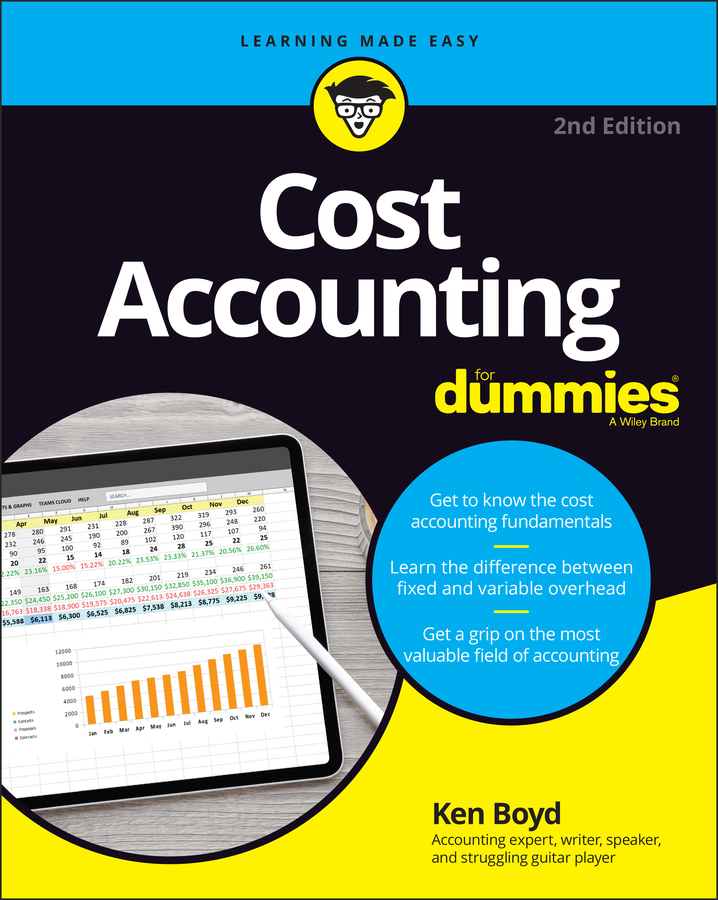##### Cost Accounting For DummiesWhen calculating depreciation of your business assets each year, you have a choice of four methods: Straight-Line, Sum-of-Years-Digits, Double-Declining Balance, and Units of Production. After you decide on the useful life of an asset and calculate its cost basis, you have to decide how to go about reducing the asset’s value according to accounting standards.

To demonstrate how the methods handle assets differently, following sections calculate the first year’s depreciation expense for a truck purchased on January 1, 2011, with a cost basis of \$25,000. The example assumes that the truck can be sold in five years for \$5,000. This is the salvage value.

## Straight-Line method

When depreciating assets using the Straight-Line method, you spread the cost of the asset evenly over the number of years the asset will be used. Straight-Line is the most common method used for depreciation of assets, and it’s also the easiest one to use.

Another advantage of this method is that you can use it for both accounting purposes and tax purposes. (If you use any of the other methods, you have to keep separate depreciation records — one for your financial reports and one for the tax man.)

The formula for calculating Straight-Line depreciation is:

(Cost of fixed asset – Salvage) ÷ Estimated useful life = Annual depreciation expense

For the truck in this example, the cost basis is \$25,000, the salvage value is \$5,000, and the IRS estimate of useful life is five years. With these figures, the calculation for finding the annual depreciation expense of this truck based on the Straight-Line depreciation method is:

(\$25,000 – \$5,000) ÷ 5 = \$4,000

Each year, the business’ Income Statement should include \$4,000 as a depreciation expense for this truck. You add this \$4,000 depreciation expense to the accumulated depreciation account for the truck. This accumulated depreciation account is shown below the truck’s original value on the Balance Sheet. You subtract the accumulated depreciation from the value of the truck to show a net asset value, which is the value remaining on the truck.

## Sum-of-Years-Digits method

If your asset loses a greater portion of its useful life in the early years, you can speed up its depreciation by using the Sum-of-Years-Digits (SYD) method. This method allows you to write off higher depreciation expenses in the earlier years of useful life and lower depreciation in later years.

One big disadvantage of writing off assets quickly is that the higher depreciation expense lowers your business’s net income.

Sum-of-Years-Digits requires a three-step calculation:

1. Find the SYD, with n representing the number of years of estimated useful life remaining as of the start of the fiscal year:

n(n+1) ÷ 2 = SYD

2. Find the applicable fraction using this formula:

n ÷ SYD = Applicable fraction

3. Calculate the depreciation expense using this formula:

(Cost – Salvage value) × Applicable fraction = Depreciation expense

To calculate the first year of the depreciation expense on the truck, this formula works like so:

SYD: 5(5+1) ÷ 2 = 15
Applicable fraction: 5 ÷ 15 = 1⁄3
Depreciation expense: \$20,000 × 1⁄3 = \$6,666.67

The depreciation expense written off during the truck’s first year of life is \$2,666.67 higher than it is when using the Straight-Line depreciation method.

## Double-Declining Balance method

The Double-Declining Balance method of depreciation allows you to write off an asset even more quickly than the Sum-of-Years-Digits method. This method is ideal for assets whose primary usefulness is in the early years of life.

You calculate the depreciation using the Double-Declining Balance method using this formula:

2 × (1 ÷ Estimated useful life) × Book value at the beginning of the year = Depreciation expense

The calculation for the truck’s depreciation expense using the Declining Balance method is:

2 × (1 ÷ 5) × \$25,000 = \$10,000

The depreciation expense for the first year of using the truck is \$10,000. The Double-Declining Balance method of depreciation reduces the value of an asset faster than the Sum-of-Years-Digits.

## Units of Production method

The Units of Production method of depreciation works well primarily in a manufacturing environment because it calculates depreciation based on the number of units produced in a year. Companies whose machinery usage varies greatly each year depending on the market and the number of units needed for sale make use of this depreciation method.

The formula for calculating depreciation using Units of Production is a two-step process:

1. Find the UOP rate using this formula:

(Cost – Salvage value) ÷ Estimated number of units to be produced during estimated useful life = UOP rate

2. Find the depreciation expense using this formula:

Units produced during the year × UOP rate = Depreciation expense

You only need to use the Units of Production depreciation method if you’re manufacturing the products you sell and if the usage of your equipment fluctuates widely from year to year.